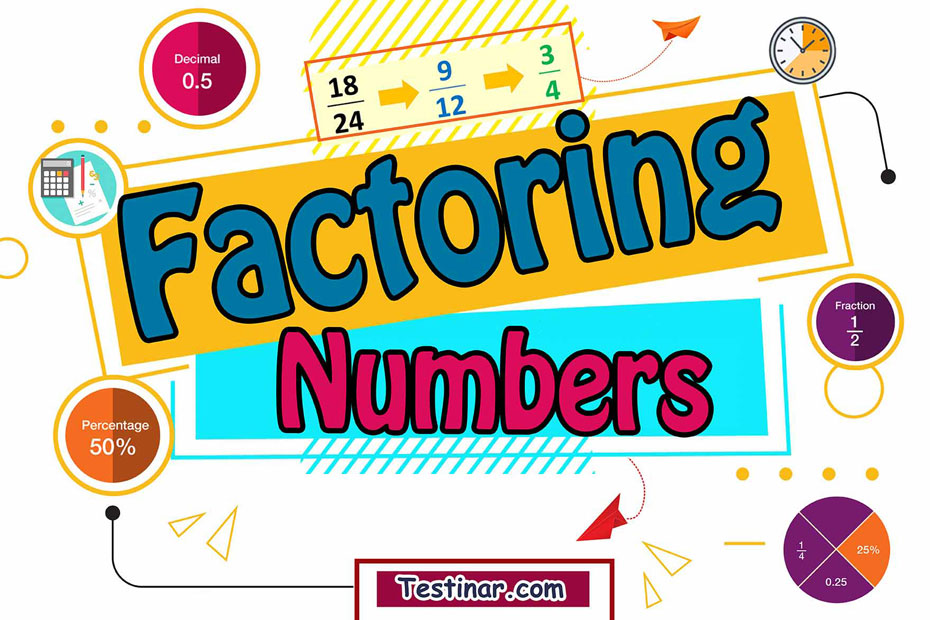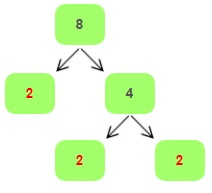## How to Factor Numbers

Whenever we multiply two whole numbers, we get another number. Now, basically, the numbers we multiplied to get the product, are called the factors of the product. So, for example, $$2 \times 3 = 6$$, this implies that $$2$$ and $$3$$ are the factors of $$6$$. Another conclusion which we can draw from this is, that factors of a number completely divide the number without leaving any remainder.

For ex: Let’s consider the number $$24$$. Now $$24$$ can be divided into factors $$6$$ and $$4$$. Also $$6$$ can be further factorized into $$3$$ and $$2$$. Moreover, $$4$$ can also be factorized into $$2$$ and $$2$$. So, from this we can see that the other factors of $$24$$ are $$3$$, $$8$$ and $$2$$. This is because $$12 \times 2 = 8 \times 3 = 3 \times 8=24$$. Now, let’s learn some facts about factors.

• Each and every number has a smallest factor which is $$1$$.
• Every number has a minimum of two factors that is $$1$$ and the number itself.
• Now, such numbers which have only two factors, i.e., $$1$$ and the number itself are called prime numbers.

#### Prime Factorization

Prime factorization is defined as the product of all the prime factors of a number which, when multiplied together, gives the original number. Moreover, sometimes to write the prime factors of a number, we may have to repeat the number. So, for example, the factors of $$8$$ are $$1$$ and $$2$$, but to represent $$8$$ we use $$8=2 \times 2 \times 2$$.How to Calculate Prime Factorization of 8?

#### Some Fun Facts about Factors

• Factors are always found to be integers and whole numbers. They can never be decimals.
• All even numbers have $$2$$ as a factor.
• Similarly, any number which end with a $$5$$, will have $$5$$ as a factor.
• Another fact is, that any integer greater than zero and that ends with a zero will always have $$2$$, $$5$$ and $$10$$ as a factor.
• Factoring is not only used in mathematics but also in algebraic expressions to simplify further.

#### How to Factor a Number

To Factor a number, follow these steps:

• Firstly, try dividing the number with $$2$$. If it isn’t divisible, try with the next prime number (i.e., $$3$$) and so on.
• Next, represent the factor as a multiple of all its prime numbers.

For example, $$42 = 2 \times 3 \times 7$$.

## How to Factor Numbers Video

### Exercises for Factoring Numbers

1) $$12 \ \Rightarrow$$

2) $$19 \ \Rightarrow$$

3) $$34 \ \Rightarrow$$

4) $$41 \ \Rightarrow$$

5) $$14 \ \Rightarrow$$

6) $$35 \ \Rightarrow$$

7) $$24 \ \Rightarrow$$

8) $$63 \ \Rightarrow$$

9) $$50 \ \Rightarrow$$

10) $$70 \ \Rightarrow$$

1) $$12 \ \Rightarrow \ \color{red}{2 \times 2 \times 3}$$
Solution
Step 1: Divide the number by the smallest prime number that is $$2$$ and continue with other prime numbers until the result is not divisible by any prime number.
$$12 \div \color{red}{2} = 6$$  ,  $$6 \div \color{red}{3} = \color{red}{2}$$
Step 2: Finally, represent the number as a product of all the prime factors. $$12 =\color{red}{2 \times 2 \times 3}$$
2) $$19 \ \Rightarrow \ \color{red}{19}$$
Solution
Step 1: The smallest prime number that $$19$$ is divisible by is  $$19$$ : $$19 \div 19 = 1$$
Step 2: Therefore $$19 = \color{red}{19}$$
3) $$34 \ \Rightarrow \ \color{red}{ 2, 17}$$
Solution
Step 1: Divide the number by the smallest prime number that is $$2$$ and continue with other prime numbers until the result is not divisible by any prime number.
$$34\div \color{red}{2} = 17$$ , $$17 \div \color{red}{17}= 1$$
Step 2: Finally, represent the number as a product of all the prime factors. $$19 = 2 \times 17$$
4) $$41 \ \Rightarrow \ \color{red}{1 \times 41}$$

5) $$14 \ \Rightarrow \ \color{red}{2 \ \times \ 7}$$

6) $$35 \ \Rightarrow \ \color{red}{5 \ \times \ 7}$$

7) $$24 \ \Rightarrow \ \color{red}{2 \times 2 \times 2 \times 3}$$

8) $$63 \ \Rightarrow \ \color{red}{3 \ \times \ 3 \ \times \ 7}$$

9) $$50 \ \Rightarrow \ \color{red}{2 \times 5 \times 5}$$

10) $$70 \ \Rightarrow \ \color{red}{2 \ \times \ 5 \ \times \ 7}$$

## Factor Numbers Quiz

### The Most Comprehensive TSI Math Preparation Bundle

$76.99$36.99

### TSI Mathematics Formulas

$6.99$5.99

### Comprehensive CLEP College Algebra Practice

$19.99$14.99

### SSAT Middle Level Math Prep 2020

$20.99$15.99